# Text Classification

Date:

In this project, we used 3 different metrics (Information Gain, Mutual Information, Chi Squared) to find important words and then we used them for the classification task. We compared the result at the end.

This project has two parts. In the first part, we find features (words which are highly related to the topics). In the second part, we classify documents, using different feature sets and evaluate the results.

# Part 1

Each document can be represented by the set of words that appear in it.
But some words are more important and has more effect for determination of the context of the document.
So we want to represent a document by a set of important words. In this part, we are going to find a set of 100 words that are more infomative for document classification.

## Data Set

The dataset for this task is “همشهری” (Hamshahri) that contains 8600 persian documents.

## Preproceesing

We did some preprocessing for building our data structures for processing.
We read each document and added its words to our vocabulary set and also a set for that document.
We then used this set to create a vocab_index dictionary for assigning an index for each word that appears in our dataset.
Our dataset consists of 5 different classes.

class_name

['ورزش', 'اقتصاد', 'ادب و هنر', 'اجتماعی', 'سیاسی']


There is some statistcs information about out dataset.

print("vocab size:", len(vocab))
print ("number of terms (all tokens):", number_of_terms)
print ("number of docs:", number_of_docs)
print ("number of classes:", cls_index)

vocab size: 65880
number of terms (all tokens): 3506727
number of docs: 8600
number of classes: 5


The probability of each classes are stored in the table below.

tmp_view = pd.DataFrame(probability_of_classess)
tmp_view

0
00.232558
10.255814
20.058140
30.209302
40.244186

# Calculation of our metrics for each words in Vocabulary We are going to find 100 words that are good indicator of classes.
We want to use 3 different type of metrics

• Information Gain
• Mutual Information
• $\chi$ square

## Information Gain

We used these metric. And the top 10 words with highest information gain can be seen in the table below.

preview.head(10)

information_gainword
00.612973ورزشی
10.516330تیم
20.297086اجتماعی
30.293313سیاسی
40.283891فوتبال
50.267878اقتصادی
60.225276بازی
70.223381جام
80.197755قهرمانی
90.177807اسلامی

If you think about the meaning of these words you can agree that since they a have high information gain they can be really good identifiers for categorizing a doc.
In the table below, you can see 5 worst words.

preview.tail(5)

information_gainword
658750.000042تبهکاران
658760.000042پذیرفتم
658770.000041توقف
658780.000031سلمان
658790.000027چهارمحال

## Mutual Information

There is two formula for this metrics. One of them is introduced in the Lecture Slides.
Another one is introduced in Website: https://nlp.stanford.edu/IR-book/html/htmledition/mutual-information-1.html
We used both of them and then choose the better set.
Let's see the result of each of those different formulae for this metric.

### First Model:

Calculating with formula in the Slides:

$MI(w, c_i) = \log{\frac{p(w, c_i)}{p(w)*p(c_i)}}$

The result is

preview.head(10)

mutual information(MI)main class MImain_classword
00.5244181.782409ادب و هنرمایکروسافت
10.5244181.782409ادب و هنرباغات
20.5244181.782409ادب و هنرنباریدن
30.5241911.703799اقتصادآلیاژی
40.5241911.703799اقتصاددادمان
50.5241911.703799اقتصادفرازها
60.5241911.703799اقتصادسیاتل
70.5216801.782409ادب و هنرسخیف
80.5216801.782409ادب و هنرکارناوال
90.5216801.782409ادب و هنرتحتانی

#### But there is a __problem__ here

The words in the table above, are infrequent words and because of that, they give use high information when they appear in a doc, but the probability of appearing such a word in any document is really small. So, in general, these words are not good identifiers.
You can see number of occurance of some of these words in each classes:

word_occurance_frequency_vs_class[word_index['نباریدن']], word_occurance_frequency_vs_class[word_index['مایکروسافت']],  word_occurance_frequency_vs_class[word_index['آلیاژی']]

(array([0, 4, 1, 0, 0]), array([0, 4, 1, 0, 0]), array([0, 5, 1, 0, 0]))


### Second Model

Now we want to calculate it with formula in this link.

The formula is:

$MI(w, c_i) = \sum_{i,j \subset \{ 0 , 1 \}} {p(w=i, c_i=j)} * \log{\frac{p(w=i, c_i=j)}{p(w=i)*p(c_i=j)}}$
mutual information(MI)main class MImain_classword
00.2026740.606665ورزشورزشی
10.1739290.512590ورزشتیم
20.0998330.279402ورزشفوتبال
30.0948180.258578سیاسیسیاسی
40.0885170.232945اقتصاداقتصادی
50.0858580.265246اجتماعیاجتماعی
60.0765820.209092ورزشبازی
70.0760980.217883ورزشجام
80.0703870.195426ورزشقهرمانی
90.0562530.155666ورزشبازیکن

#### These words are better

They are not rare
You can see the frequency of some of these words in each classes:

print (list(reversed(class_name)))
word_occurance_frequency_vs_class[word_index['ورزشی']], word_occurance_frequency_vs_class[word_index['سیاسی']],  word_occurance_frequency_vs_class[word_index['پیروزی']],

['سیاسی', 'اجتماعی', 'ادب و هنر', 'اقتصاد', 'ورزش']

(array([1866,    9,    8,   66,   16]),
array([  35,  300,   71,  372, 1602]),
array([497,  44,  24,  60, 191]))


## $\chi$ Squared

Another Metric is $\chi$ Squared.
Now we are goind to see the result of using $\chi$ squared as a mesure of importance.

chi squaredmain class chimain_classword
02234.5916007376.515420ورزشورزشی
12028.1869596701.136193ورزشتیم
21302.9478034296.622009ورزشفوتبال
31050.2892813459.737651ورزشجام
41043.4789413138.957039سیاسیسیاسی
51029.4814513055.759330اقتصاداقتصادی
61027.3111673254.137538ورزشبازی
7967.9232543299.353018اجتماعیاجتماعی
8961.0787693169.912598ورزشقهرمانی
9772.0412052558.041173ورزشبازیکن

## Result Comparison

We can compare our three set of words here.
In the table below, you can see top 20 words for each metrics.
For mutual information, there are two sets because we used two different formula.

information gain1chi squared1mutual information_model_2mutual information_model_1
0ورزشیورزشیورزشیمایکروسافت
1تیمتیمتیمباغات
2اجتماعیفوتبالفوتبالنباریدن
3سیاسیجامسیاسیآلیاژی
4فوتبالسیاسیاقتصادیدادمان
5اقتصادیاقتصادیاجتماعیفرازها
6بازیبازیبازیسیاتل
7جاماجتماعیجامسخیف
8قهرمانیقهرمانیقهرمانیکارناوال
9اسلامیبازیکنبازیکنتحتانی
10بازیکنبازیکناناسلامیمعراج
11مجلسفدراسیونبازیکنانخلافت
12بازیکنانمسابقاتفدراسیوننجیب
13فدراسیوندلارمسابقاتزبون
14مسابقاتقیمتدلارصحیفه
15شورایمسابقهمسابقهمشمئزکننده
16مسابقهآسیامجلسارتجاع
17دلارگذاریقیمتذبیح
18آسیاصنایعشورایوصنایع
19مردمسرمایهگذاریتوپخانه

## Output File

The output files are stored in CSV format files.
These files contain 100 most important words for each metrics.

## Conclusion of Part 1

When you look at the last table you can see first three columns are similar to each other and have nearly same words but last columns’ (mutual information with formula 1) words are different from other columns
We can conclude that the Formula 1 has different behavior and probably is not efficient. So it is better to use formula 2 for calculating Mutual Information

For more accurate comparisons on which of these three metrics are better, we can test it.

# Part 2

In Part 1 we tried to find good features to vectorize documents. We used three metrics and extracted three set of 100 words.
Each document can be represented by the set of words that appear in the document.
In this part we want to use these sets of features to classify documents with SVM.

## Evaluation

For evaluating our classification we used k-fold cross-validation with k=5.
We reported our average of these 5 confusion matrices.

## Vectorizing Documents We wanted to vectorize our documents. We did this with 4 different methods:

1) Using 1000 most frequent words as features set
2) Using Information Gain features
3) Using Mutual Information features
4) Using $\chi$ square features

## Storing word frequencies

We need to store word frequency in each document for the processing

## 1) Using 1000 most frequent words as feature set

There is an ambiguity in defining the meaning of frequent.

• First meaning: A word is frequent if in lots of document there is at least one occurrence of this word.
• Second meaning: A word is frequent if the sum of the number of occurrence of this word in all documents is high. (Maybe in one document there are lots of occurrences but in another document, there is no occurrence.)

In this code, we chose the first meaning.

01
08415و
18352در
28241به
37956از
47838این
57382با
67240که
76923را
86912است
96859می

The above words are 10 most frequent words in our dataset. And all of them are stop words.

### Making Vector X

We want to make the vector for each document and then use this vectors for classification.
We used our 1000 words for vectorizing.

### Using SVM for classification

We used svm classifier for our classification.

### Confusion Matrix for 1000 most frequent

print ("accuracy:", first_method_accuracy,'\n')
print ("confusion matrix:\n")
first_method_cm = confusion_matrix_avg
pd.DataFrame(first_method_cm)

accuracy: 0.8787209302325582

confusion matrix:

01234
0389.02.20.06.42.4
10.2414.20.26.419.0
21.210.837.244.26.6
31.618.41.6300.637.8
41.019.20.029.4370.4

## 2) Using 100-dimensional vector with Information Gain

information_gainword
00.612973ورزشی
10.516330تیم
20.297086اجتماعی
30.293313سیاسی
40.283891فوتبال
50.267878اقتصادی
60.225276بازی
70.223381جام
80.197755قهرمانی
90.177807اسلامی

### Making Vector X

We want to make vector for each document and then use this vectors for classification

### Using SVM for classification

print ("accuracy:", second_method_accuracy,'\n')
print ("confusion matrix:\n")
second_method_cm = confusion_matrix_avg
pd.DataFrame(second_method_cm)

accuracy: 0.806279069767442

confusion matrix:

01234
0376.07.60.211.44.8
11.4382.60.222.033.8
24.024.07.255.09.8
32.430.81.0268.857.0
42.431.60.233.6352.2

## 3) Using 100-dimensional vector with Mutal Information

We used the better formula for selecting 100 words.

features.head(10)

mutual informationmain class scoremain classword
00.2026740.606665ورزشورزشی
10.1739290.512590ورزشتیم
20.0998330.279402ورزشفوتبال
30.0948180.258578سیاسیسیاسی
40.0885170.232945اقتصاداقتصادی
50.0858580.265246اجتماعیاجتماعی
60.0765820.209092ورزشبازی
70.0760980.217883ورزشجام
80.0703870.195426ورزشقهرمانی
90.0562530.155666ورزشبازیکن

### Making Vector X

We want to make vector for each document and then use this vectors for classification

### Using SVM for classification

print ("accuracy:", third_method_accuracy,'\n')
print ("confusion matrix:\n")
third_method_cm = confusion_matrix_avg
pd.DataFrame(third_method_cm)

accuracy: 0.804186046511628

confusion matrix:

01234
0375.28.20.011.45.2
11.4379.80.222.636.0
23.823.07.056.49.8
32.031.41.0270.055.6
42.431.20.235.0351.2

## 4) Using 100-dimensional vector with $\chi$ Squared

features.head(10)

chi squaredmain class scoremain classword
02234.5916007376.515420ورزشورزشی
12028.1869596701.136193ورزشتیم
21302.9478034296.622009ورزشفوتبال
31050.2892813459.737651ورزشجام
41043.4789413138.957039سیاسیسیاسی
51029.4814513055.759330اقتصاداقتصادی
61027.3111673254.137538ورزشبازی
7967.9232543299.353018اجتماعیاجتماعی
8961.0787693169.912598ورزشقهرمانی
9772.0412052558.041173ورزشبازیکن

### Making Vector X

We want to make vector for each document and then use this vectors for classification

### Using SVM for classification

print ("accuracy:", forth_method_accuracy,'\n')
print ("confusion matrix:\n")
forth_method_cm = confusion_matrix_avg
pd.DataFrame(forth_method_cm)

accuracy: 0.8025581395348838

confusion matrix:

01234
0375.28.20.011.45.2
11.4380.80.222.235.4
23.825.06.655.69.0
32.431.20.8267.258.4
42.431.80.235.0350.6

## Comparision

We compare our result with these 4 methods with confusion matrix and accuracy
The result is as follow

preview = pd.DataFrame({'1000words': [first_method_accuracy],
'InfoGain': [second_method_accuracy],
'mutual info': [third_method_accuracy],
"chi squared": [forth_method_accuracy]})
print ("accuracy:")
preview

accuracy:

1000wordsInfoGainchi squaredmutual info
00.8787210.8062790.8025580.804186
preview = pd.concat([pd.DataFrame(first_method_confusion_matrix),
pd.DataFrame(second_method_confusion_matrix),
pd.DataFrame(third_method_confusion_matrix),
pd.DataFrame(forth_method_confusion_matrix)], axis=1)
print ("confusion matrix:")
print ("\t1000 words\t\t\t IG \t\t \t MI \t\t   chi squared")
preview

confusion matrix:
1000 words			 IG 		 	 MI 		   chi squared

01234012340123401234
0389.02.20.06.42.4376.07.60.211.44.8375.28.20.011.45.2375.28.20.011.45.2
10.2414.20.26.419.01.4382.60.222.033.81.4379.80.222.636.01.4380.80.222.235.4
21.210.837.244.26.64.024.07.255.09.83.823.07.056.49.83.825.06.655.69.0
31.618.41.6300.637.82.430.81.0268.857.02.031.41.0270.055.62.431.20.8267.258.4
41.019.20.029.4370.42.431.60.233.6352.22.431.20.235.0351.22.431.80.235.0350.6

## Visualization

We are going to show each one in separated tables:


plt.figure()
plot_confusion_matrix(first_method_cm, classes=class_name,
title='Confusion matrix visualization for 1000 most frequent_normalized', normalize=True);
plt.show()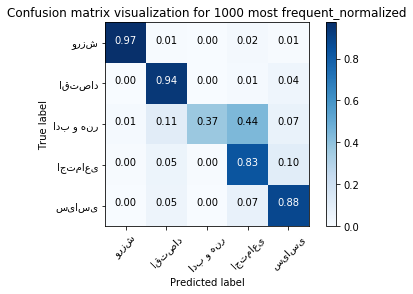plt.figure()
plot_confusion_matrix(second_method_cm, classes=class_name,
title='Confusion matrix visualization for Information gain_normalized', normalize=True);
plt.show()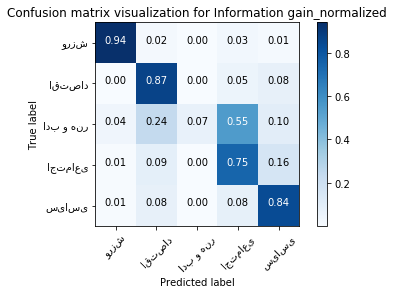plt.figure()
plot_confusion_matrix(third_method_cm, classes=class_name,
title='Confusion matrix visualization for Mutual information_normalized', normalize=True);
plt.show()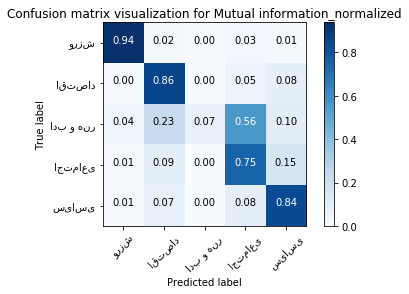plt.figure()
plot_confusion_matrix(forth_method_cm, classes=class_name,
title='Confusion matrix visualization for chi squared_normalized', normalize=True);
plt.show()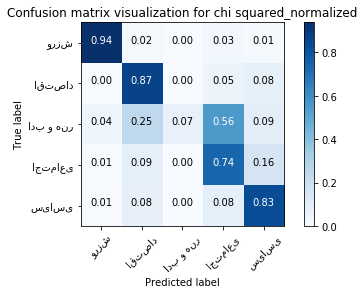#### Now we want to test the result for most 100 frequent word vector

print ("accuracy:", first_method_accuracy_100,'\n')
print ("confusion matrix:\n")
first_method_cm_100 = confusion_matrix_avg
pd.DataFrame(first_method_cm_100)

accuracy: 0.803953488372093

confusion matrix:

01234
0375.68.20.011.44.8
11.4382.40.422.033.8
23.224.87.255.29.6
31.830.81.0267.459.0
42.432.60.234.6350.2
plt.figure()
plot_confusion_matrix(first_method_confusion_matrix_100, classes=class_name,
title='Confusion matrix visualization for 100 most frequent_normalized', normalize=True);
plt.show()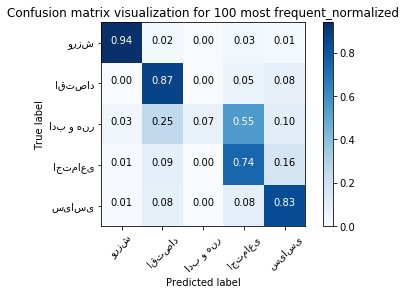## Conclusion

We know that 1000 words are not best words because these words include stop words that they are not informative. But because they are 1000 words rather than 100 words, the result is going to be better.
We test a set of 100 most frequent words. The result was acc = 0.8049 which is similar to other three methods. We also show the confusion matrix for 100 most frequent in the last table above. And you can see this table is also similar to other three ones
So we can guess that there is no significant difference between choosing these metrics for selecting words in document classification task.
And we also know that Information Gain doesn’t store every class information gains (We only stored one number Information Gain for every word). But we can consider each class information gain if we split the Sigma over classes in information gain formula.(And consider the meaning of Entropy for each class). So it has the same functionality as other metrics.
We guess that if the dimension of our vector increase we probably are going to get more accuracy.
And also maybe if we use different features depending on the sequence of words that appear each document, we can get more accuracy.